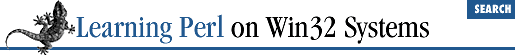home | O'Reilly's CD bookshelfs | FreeBSD | Linux | Cisco | Cisco Exam

### 4.6 The foreach Statement

Yet another iteration construct is the ``` foreach``` statement. This statement takes a list of values and assigns them one at a time to a scalar variable, executing a block of code with each successive assignment. It looks like this:

```foreach ```

\$i
```
(```

@some_list
```
) {
```

statement_1
```
;
```

statement_2
```
;
```

statement_3
```
;
}```

The original value of the scalar variable is automatically restored when the loop exits; another way to say this is that the scalar variable is local to the loop.

Here's an example of a ``` foreach``` :

```@a = (1,2,3,4,5);
foreach \$b (reverse @a) {
print \$b;
}```

This program snippet prints ``` 54321``` . Note that the list used by the ``` foreach``` can be an arbitrary list expression, not just an array variable. (This flexibility is typical of all Perl constructs that require a list.)

You can omit the name of the scalar variable, in which case Perl pretends you have specified the ``` \$_``` variable name instead. You'll find that the ``` \$_``` variable is used as a default for many of Perl's operations, so you can think of it as a scratch area.[ 4 ] (All operations that use ``` \$_``` by default can also use a normal scalar variable as well.) For example, the ``` print``` function prints the value of ``` \$_``` if no other value is specified, so the following example works like the previous one:

 Which means you'd better localize it in functions when we learn about them.

```@a = (1,2,3,4,5);
foreach (reverse @a) {
print;
}```

See how using the implied ``` \$_``` variable makes it easier? After you've learned more functions and operators that default to ``` \$_``` , this construct will become even more useful. This is one case where the shorter construct is more legible than the longer one.

If the list you are iterating over is made of real variables rather than some function returning a list value, then the variable being used for iteration is in fact an alias for each variable in the list instead of being merely a copy of the values. Consequently, if you change the scalar variable, you are also changing that particular element in the list that the variable is standing in for. For example:

```@a = (3,5,7,9);
foreach \$one (@a) {
\$one *= 3;
\$x = 17;
@a = (3,5,7,9);
@b = (10,20,30);
foreach \$one (@a, @b, \$x) {
\$one *= 3;
}
# \$x is now 51
# @a is now (9,15,21,27)
# @b is now (30,60,90);
}
# @a is now (9,15,21,27)```

Notice how altering ``` \$one``` in fact altered each element of ``` @a``` .4.5 The for Statement4.7 Exercises